# 单智能体强化学习算法

## Diversity is All You Need：Learning Skills without a Reward Function

Posted by MY on September 29, 2020

## 二、解法

#### 2.1 优化目标设计

• 不同的skill对应于不同的状态空间，相当于探索不同的区域，从而使得skill是distinguishable的
• 通过状态而不是动作来区分skill，因为许多动作可能导致环境发生相同的转移；同时通过当前的状态，模型应该能够轻松的推断出当前的skill是什么
• 让skill尽可能diverse，尽可能随机的act，但始终要保持distinguishable

DIYAN中，这种学习目标表述为“最大化状态$s$和对应skill $z$的互信息”。具体的，DIYAN最大化的目标如下：

$\mathcal{F}(\theta)=I(S ; Z)+H(A \mid S)-I(A ; Z \mid S)$

• 第一项是$S$和$Z$的互信息，是目标函数的核心
• 第二项是将所有skill混合起来的mixed policy的熵，本文希望动作具有多样性，这也是RL中常用的目标，如A3C, SAC。
• 第三项是在已知状态$S$的情况下， $Z$和$A$的互信息。DIYAN希望$Z$和状态$S$的关系密切，同时尽量减少在已知状态下$Z$和动作$A$的关系，强调不能以动作来区分skill

\begin{aligned} \mathcal{F}(\theta) & \triangleq I(S ; Z)+\mathcal{H}[A \mid S]-I(A ; Z \mid S) \\ &=(\mathcal{H}[Z]-\mathcal{H}[Z \mid S])+\mathcal{H}[A \mid S]-(\mathcal{H}[A \mid S]-\mathcal{H}[A \mid S, Z]) \\ &=\mathcal{H}[Z]-\mathcal{H}[Z \mid S]+\mathcal{H}[A \mid S, Z] \\ &=H(A \mid S, Z)+\mathbb{E}_{s \sim \pi(z), z \sim p(z \mid s)}[\log p(z \mid s)]-\mathbb{E}_{z \sim p(z)}[\log p(z)] \end{aligned}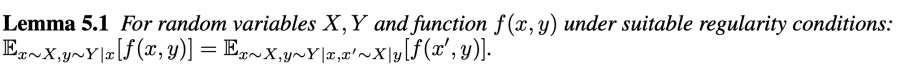$\mathcal{F}(\theta)=H(A \mid S, Z)+\mathbb{E}_{s \sim \pi(z), z \sim p(z)}[\log p(z \mid s)]-\mathbb{E}_{z \sim p(z)}[\log p(z)]$

\begin{aligned} \mathcal{F}(\theta) &=H(A \mid S, Z)+\mathbb{E}_{s \sim \pi(z), z \sim p(z)}\left[\log \frac{p(z \mid s)}{q(z \mid s)}+\log q(z \mid s)\right]-\mathbb{E}_{z \sim p(z)}[\log p(z)] \\ &=H(A \mid S, Z)+D_{K L}[p(z \mid s) \| q(z \mid s)]+\mathbb{E}_{s \sim \pi(z), z \sim p(z)}[\log q(z \mid s)]-\mathbb{E}_{z \sim p(z)}[\log p(z)] \\ & \geq H(A \mid S, Z)+\mathbb{E}_{s \sim \pi(z), z \sim p(z)}[\log q(z \mid s)-\log p(z)] \end{aligned}

#### 2.2 算法实现

DIYAN使用SAC训练，SAC本身在策略优化过程中，包括了对policy entropy的maximization，也就是对应优化目标中的第一项。我们仅考虑后面两项$\mathbb{E}_{s \sim \pi(z), z \sim p(z)}[\log q(z \mid s)-\log p(z)]$。由于$p(z)$一般是一个固定的分布，因此关键在于最大化$\mathbb{E}[\log q(z \mid s)]$。

• 使用神经网络$q_{\phi}(z \mid s)$代表该概率分布，输入为$s$，输出为隐变量$z$。神经网络的参数为$\phi$。对于交互过程中采样的序列$\left(s_{1}, z_{1}, a_{1}\right),\left(s_{2}, a_{2}, z_{2}\right), \ldots$, 直接使用监督学习的方式，来最大化输出$z$的似然。例如当 latent 是离散变量时，该监督学习问题是一个标准的分类问题。
• 强化学习使用该目标函数构造的奖励来训练策略，需要最大化的项可以设置为奖励的形式。期望可以使用蒙特卡罗方法来采样进行估计。奖励为$r_{z}(s, a) \triangleq \log q_{\phi}(z \mid s)-\log p(z)$。该奖励的直观含义是，agent应该按照这种最大化累积该奖励的方式更新policy/skill，使得agent在特定skill下到达的state能够容易地推断出对应的skill。该奖励使用SAC来最大化。

#### 2.3 算法流程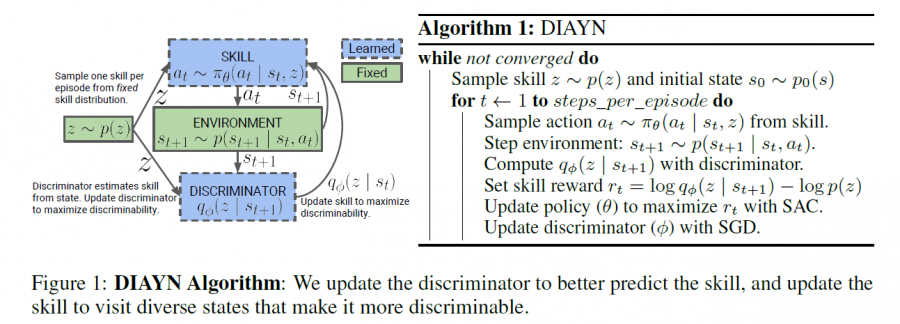## 三、实验内容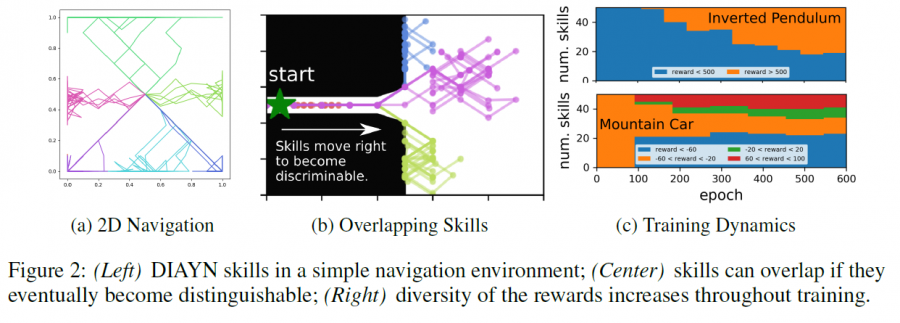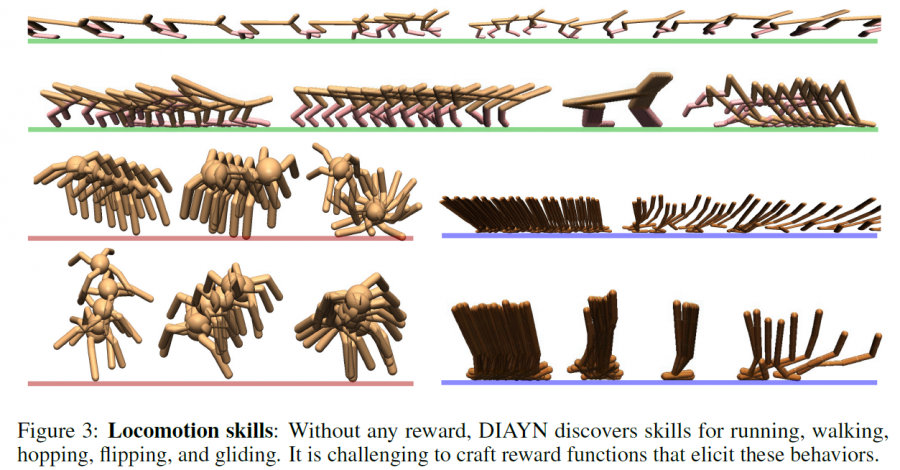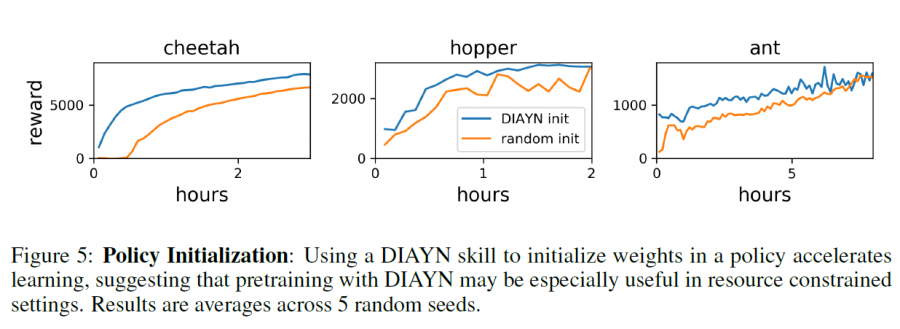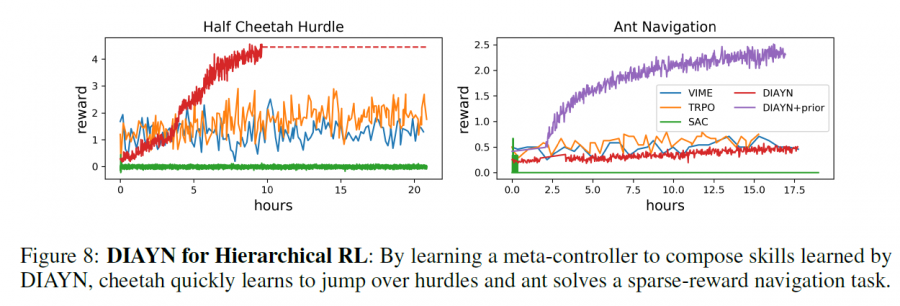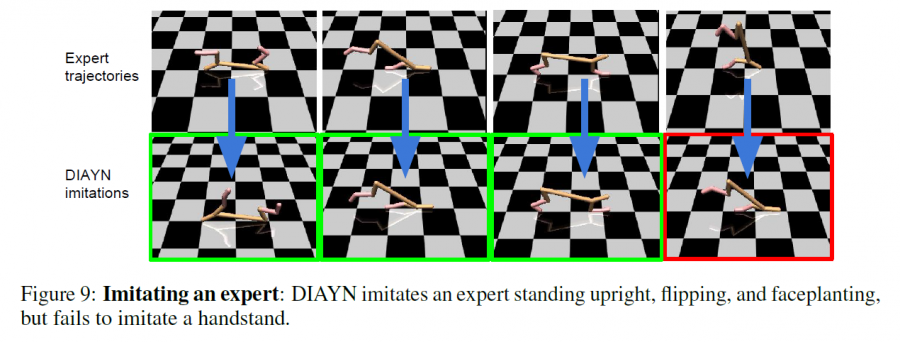## 五、优点

### 参考

##### Share